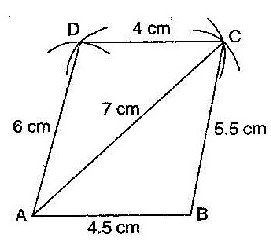# Construct the quadrilateral ABCD. AB is equal to 4.5 cm, BC is equal to 5.5 cm, CD is equal to 4 cm, AD is equal to 6 cm and AC is equal to 7 cm

Given: AB is equal to 4.5 cm, BC is equal to 5.5 cm, CD is equal to 4 cm, AD is equal to 6 cm and AC is equal to 7 cm

Use the following steps:
• Draw a line segment AB=4.5cm
• Take B as center and draw an angle of 60 o
• Cut off BC=5.5cm
• Take A as with radius 4.8cm and draw an arc.
• Take C as with radius 5cm and draw an arc, which cuts off previous arc at point DUpdated on: 10-Oct-2022

39 Views